## Solution to 1986 Problem 96

 The first-order correction to the nondegenerate state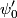$\psi_0'$ in time-independent nondegenerate perturbation theory is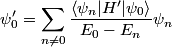\begin{align*}\psi_0' = \sum_{n \neq 0} \frac{\langle \psi_n | H' | \psi_0 \rangle}{ E_0 - E_n} \psi_n\end{align*}where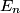$E_n$ is the energy of the unperturbed state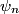$\psi_n$. In this case,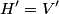$H' = V'$, which is an even function.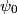$\psi_0$ is also an even function. Therefore, whenever,$\psi_n$ is odd, the inner product will be zero. Therefore, answer (B) is correct.